# DAV Class 6 Maths Chapter 13 Brain Teasers Solutions

The DAV Maths Book Class 6 Solutions and DAV Class 6 Maths Chapter 13 Brain Teasers Solutions of Circles offer comprehensive answers to textbook questions.

## DAV Class 6 Maths Ch 13 Brain Teasers Solutions

Question 1.
A. Tick (✓) the correct answer.
(a) Plane figure which is not made of any line segment is called
(i) square
(ii) triangle
(iii) circle
(iv) rectangle
(iii) circle.
Square, triangle and rectangle are plane figures. They are made of line segment. Circle is also a plane figure but it is not made of line segment. It is made of curved line.

(b) Number of diameters that can be drawn in a circle:
(i) one
(ii) two
(iii) many
(iv) three
(iii) many.
One can draw many diameters in a circle.

(c) In the given figure if X is the centre of the circle, then AXYZ is(i) isosceles
(ii) equilateral
(iii) right
(iv) scalene
(i) isosceles.
Isosceles triangle has equal two sides.

(d) In the given figure if radius of inner circle is 2 cm, the diameter of the outer circle will be(i) 4 cm
(ii) 8 cm
(iii) 6 cm
(iv) 10 cm
(ii) 8 cm.
Radius of inner circle = 2 cm.
∴ Diameter of inner circle = 2 × 2 cm = 4 cm
∴ Radius of outer circle = 4
Now, the diameter of outer circle = 2 × 4 cm = 8 cm.

(e) In the given figure, O is the centre of circle, then(i) PQ and RS are always equal.
(ii) PQ and RS are never equal.
(iii) PQ and RS may or may not be equal.
(iv) PQ is greater than RS.
(i) PQ and RS are always equal
ΔPOQ ≅ ΔROS.
That is why all the corresponding sides are equal.(a) If length of a chord passing through the centre of the circle is 10 cm, what is the radius of the circle?
5 cm.
As the chord is passing through the centre of the circles, it is a diameter whose length is 10 cm.
Thus, radius r = $$\frac{d}{2}=\frac{10}{2}$$ = 5cm.

(b) What will be the width of the following circular race track with inner diameter 10 cm and outer diameter 14 cm.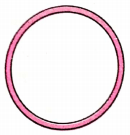2 cm.
Outer diameter = 14 cm
ourter radius = $$\frac{14}{2}$$ = 7 cm
Inner diameter = 10 cm
inner radius = $$\frac{10}{2}$$ = 5 cm
Width of circular race track = 7 cm – 5 cm = 2 cm

(c) If two circles of centres O and C with radii 2 cm and 3 cm touch externally, what is the distance between their centres?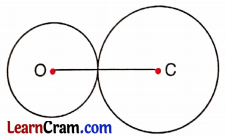5 cm.
The distance between the two circles.
= r1 + r2 = 2 + 3 = 5 cm

(d) In the following figure O is the centre of the circle with OP = 4 cm. Find QR.8 cm.
Here OP = OQ = OR = OS = 4 cm
QR = OQ + OR = 4 cm + 4 cm = 8 cm

(e) In the following figure name the line segments of equal length, where O is the centre of the circle.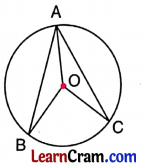OA = OB = OC.
OA, OB and OC are radius of the same circle which is equal.Question 2.
Construct a circle of any radius and draw the following in it.
(a) Centre 0
(b) Diameter AB
(d) Chord AY
(e) Any arc XYZ.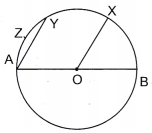Steps:
(a) Mark any point O on your paper sheet as a centre.
(b) Draw a line segment through O whose end points meet the circle at A and B. AB is the required diameter.
(c) Take any point X on the circle. Join OX. OX is the required radius of the circle.
(d) Take any other point Y on the circle. Join AY. AY is the required chord of the circle.
(e) Take any point Z between A and Y on the circle. XYZ is the required arc of the circle.

Question 3.
Draw a circle of radius 4.2 cm. Draw its diameter and measure it.Steps:
(i) Draw a circle with radius 4.2 cm with O as the centre of the circle.
(ii) Draw a line segment through O whose end points A and B lie on the circle.
(iii) Measure the diameter AB with the help of scale. The length of AB is 8.4 cm.Question 4.
Draw a line segment PQ of length 7 cm. At P, draw a circle of radius 4 cm. At Q, draw a circle of radius 3 cm. What do you observe?(i) Draw a line segment PQ = 7 cm.
(ii) Draw a circle of radius 4 cm and centre P.
(iii) Draw another circle with centre Q and radius 3 cm.
(iv) We observe that the two circles touch each other at R.
∵ 4 cm + 3 cm = 7 cm

Question 5.
Draw a line segment XY of length 8 cm. At X, draw a circle of radius 5 cm. At Y, draw a circle of radius 3.8 cm. What do you observe?Steps:
(i) Draw XY = 8 cm.
(ii) Draw a circle with centre X and radius 5 cm.
(iii) Draw another circle with centre Y and radius 3.8 cm.
(iv) We observe that the two circles intersect each other at A and B.
∵ 5 cm + 3.8 = 8.8 cm > 8 cm.

Question 6.
Write ‘True’ or ‘False’ for the following statements.
(a) All chords of a circle are of equal length.
False

(b) If diameter of a circle is 3 cm, its radius is 6 cm.
False

(c) Diameter is the longest chord of a circle.
True

(d) Semi-circle is an arc of the circle.
True

(e) The centre of a circle lies in the interior of the circle.
TrueQuestion 7.
Look at the circle carefully and answer the questions.
(a) Name two diameters.
(b) How many chords are there?
(d) Name any two arcs.
(e) Name one semi-circle.
(g) Is CX a chord?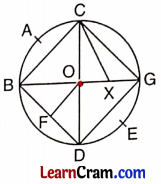(a) CD and BG, are two diameters.
(b) There are 6 chords.
(c) OC, OB and OD are the radii of the circle.
(d) Arc DEG and arc BAC.
(e) Semicircle CGED.
(f) No, FO is not a radius.
(g) No, CX is not a chord.

### DAV Class 6 Maths Chapter 13 HOTS

Question 1.
If the circumference of a circle with radius 3.5 cm and centre 0 is 22 cm. Find the perimeter of the quarter of the circle.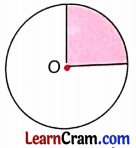Here circumference = 22 cm.
R = 3.5 cm.
Perimeter of the quarter of the circle
= $$\frac{1}{4}$$ × 22 + R + R
= $$\frac{1}{4}$$ × 22 + 3.5 + 3.5 cm
= $$\frac{1}{4}$$ × 22 + 7 cm
= 5.5 cm + 7 cm
= 12.5 cm.

Question 2.
A wire is in the shape of a square of side 4 cm which is bent to form a circle. What will be the circumference of the circle?
Perimeter of square = 4 × side
= 4 × 4 cm = 16 cm
Now, perimeter of square = circumference of the circle = 16 cm.
Thus, circumference of circle = 16 cm.

Question 1.
The diameter of a circle is 10.5 cm, find its radius.
Radius = $$\frac{1}{2}$$ × diameter
= $$\frac{1}{2}$$ × 10.5
= 5.25 cmQuestion 2.
From the given figure, answer the following questions.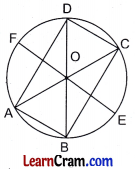(a) Number of diameters. Name them.
Number of diameters = 3, their names are AC, BD and EF.

(b) Number of chords.
Number of chords are 7.

Name of all radii are OA, OB, OE, OC, OD and OF.

(d) Number of arcs. Name any two arcs.
Number of arcs = 14, Name of two arcs are AFD and BEC.

Question 3.
Write ‘True’ or False for the following statements.
(a) The line segment joining any two points on the circle is called diameter.
False

(b) Every chord of a circle passes through the centre.
False

(c) Diameter is the longest chord of the circle.
True

(d) Diameter divides the circle in two equal parts.
True

(e) The line segment joining any two points on the circle is called a chord.
True

(f) Diameter does not pass through the centre of the circle.
False

(g) Any chord divides the circle in two arcs.
TrueQuestion 4.
Fill in the blanks:
(a) Half of the circle is called ________.
semicircle

(b) ________ is the longest chord of a circle.
Diameter

(c) Radius is of the length of diameter.
Half

(d) Circle has only ________ centre.
One

(e) Any chord divides the circle in ________ and ________ arcs.
Major, minor

(f) Diameters of different circles are ________.
different

(g) If radius of a circle is 3.2 cm then the length of the diameter is ________
6.4 cm

(h) The two diameters perpendicular to each other divide the circle into ________ equal parts.
4

(i) Perimeter of a circle is called ________.
circumference

(j) Number of diameters that can be drawn in a circle is ________.